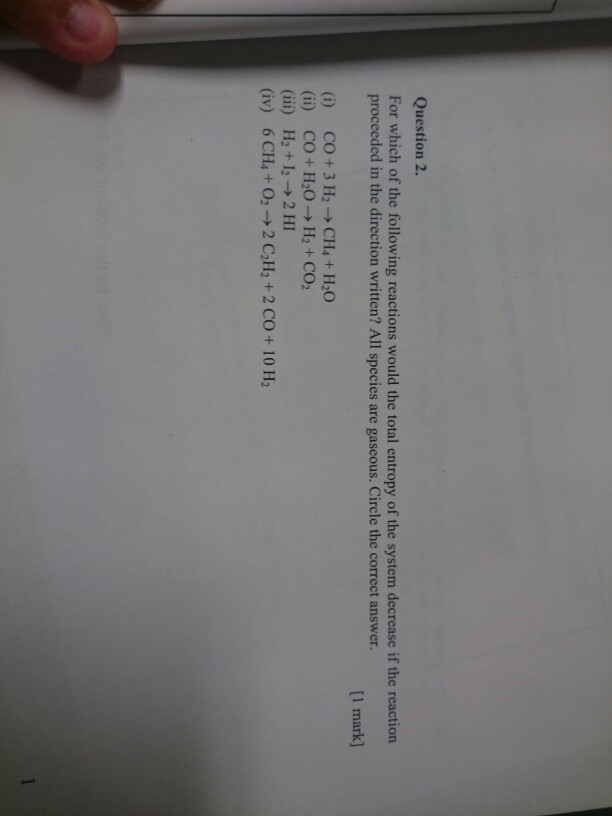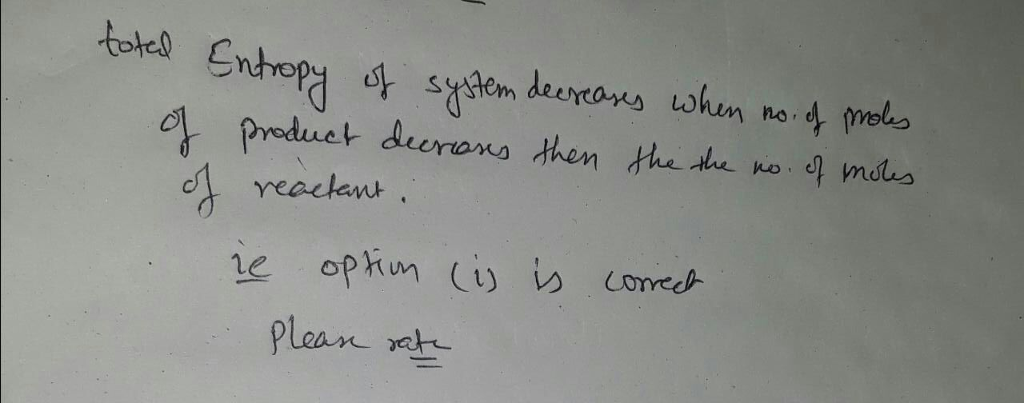# Question & Answer: For which of the following reactions would the total entropy of the system decrease if the…..For which of the following reactions would the total entropy of the system decrease if the reaction proceeded in the direction written? All species are gaseous. Circle the correct answer. (i) CO + 3 H_2 rightarrow CH_4 + H_2O (ii) CO + H_2O rightarrow H_2 + CO_2 (iii) H_2 + I_2 rightarrow 2 HI (iv) 6 CH_4 + O_2 rightarrow 2 C_2H_2 + 2 CO + 10H_2